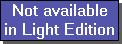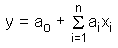The SDL Component Suite is an industry leading collection of components supporting scientific and engineering computing. Please visit the SDL Web site for more information....## RidgeRegUnit: SDL_math2 Class: none Declaration: function RidgeReg (InData: TMatrix; OutData: TVector; Lambda: double; Coeff: TVector; DeltaCoeff: TVector; var NearSingular: boolean): boolean;

Ridge regression is a variant of MLR which provides a solution to problems caused by collinear predictors xi in the MLR equation:If variables are highly correlated the estimated parameters will be unstable and tend to become extensively large. In order to reduce this unwanted effect ridge regression introduces a penalizing parameter Lambda which forces the estimated parameters to become smaller. Please note that the optimum value of the parameter Lambda has to determined by cross validation (in most cases the optimum value will be in the range between zero and 0.1).

The matrix InData contains the values of the independent variables xi, the vector OutData contains the values of the dependent variable y.

The function returns TRUE if the result is valid. In this case the coefficients a1 to an of the solution are the vector elements Coeff to Coeff[n], respectively. The constant term a0 is contained in Coeff[n+1].

Numerical instabilities which may arise from near-singular equations are indicated by returning a TRUE value in the variable parameter NearSingular. In this case the calculated coefficients should not be used.

The vector DeltaCoeff reflects the uncertainties of the estimated parameters in vector Coeff. In order to get the standard deviation of the parameters, DeltaCoeff has to be multiplied by the standard error of the residuals. The standard error can be calculated bywith n = number of rows of InData, k = number of columns of InData, and the Yi being the actual and the estimated OutData.

 Hint 1: RidgeReg uses singular value decomposition to obtain the solution.

 Hint 2: The vectors Coeff and DeltaCoeff are automatically adjusted to the required size (i.e. the number of independent variables plus 1).

Last Update: 2013-Apr-10